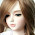# System Analysis and Design Set 6

## Questions 51 to 60

51.
Which of the statement(s) is/are correct related to role/rolenames in associations?
I.       When one class associates with another, each one usually plays a role within that association.
II.     A Role name may be placed on one or both ends of an association line.
III.   If Company and Person are two classes in a class diagram that has an association relationship, One could use an association name called “employs” or the role names of “Employer” and “Employee” to convey an employment relationship.
 (a) Only (I) above (b) Both (I) and (II) above (c) Both (I) and (III) above (d) Both (II) and (III) above (e) All (I), (II) and (III) above.
52.
Some questions related to UML followed by possible answers are given below.
I.       What does it mean for a Use Case to include another Use Case? Including a Use Case means that one Use Case incorporates the steps of another Use Case.
II.     How do you represent an object’s lifeline in a sequence diagram? The lifeline is a dashed line descending from an object that represents the existence over time of An object.
III.   Which of the following UML diagrams give a static view of a system? Class, Object, Use Case, State, Sequence Only Class, Object and Use Case Diagrams.
Which of the above question-answer combinations is/are valid?
 (a) Only (III) above (b) Both (I) and (II) above (c) Both (I) and (III) above (d) Both (II) and (III) above (e) All (I), (II) and (III) above.
53.
Which of the following shows the top level function defined to cover the scope of the application?
 (a) Context diagram (b) Decision table (c) Data flow diagram (d) Flow charting (e) Psuedocode.
54.
Which of the following is/are not considered as benefit(s) of prototyping?
 (a) Provide rapid feedback from the user to the designer (b) Help validate requirements with fewer errors (c) Enhance designer and user understanding of what system should accomplish (d) System testing can be automated (e) All of the above.
55.
A data store is represented in data flow diagram as
 (a) Rectangle (b) Square (c) Open rectangle (d) Open square (e) Ellipse.
56.the symbol represents
 (a) Aggregation (b) Generalization (c) Dependency (d) Composition (e) Association.
57.
Whole Part or Composition relationship represents
 (a) Aggregation (b) Inheritance (c) Polymorphism (d) Stereotype (e) Association.
58.
Which of the following represents a person or palce or thing about which some information is gathered?
 (a) Entity (b) Attribute (c) Data Store (d) Data flow (e) Data dictionary.
59.
Who is not involved in Requirements definition?
 (a) Client managers (b) System end-users (c) Client engineers (d) Contractor managers (e) Software developers.
60.
Which cohesion operates on the same input or output data?
 (a) Communicational (b) Temporal (c) Procedural (d) Functional (e) Object.

1.